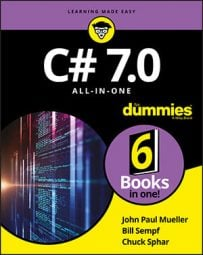##### C# 7.0 All-in-One For DummiesC# variables that contain single values are plenty useful. Even class structures that can describe compound objects made up of parts are critical. But you also need a construct for holding a bunch of objects.The built-in class `Array` is a structure that can contain a series of elements of the same type.

Given an array of strings, the following loop averages their lengths:

`public class Student // Read about classes in Book II.`

`{`

`public string name;`

`public double gpa; // Grade point average`

`}`

` `

`public class Program`

`{`

`public static void Main(string[] args)`

`{`

`// . . .create the array somehow . . .`

` `

`// Now average the students you have.`

`double sum = 0.0;`

`<strong> </strong>for (int i = 0; i < students.Length; i++)`

`{`

`sum += students[i].gpa;`

`}`

` `

`double avg = sum / students.Length;`

`// . . .do something with the average . . .`

`}`

`}`

The `for` loop iterates through the members of the array. (Yes, you can have arrays of any sort of object, not just of simple types such as `double` and `string`.) `students.Length` contains the number of elements in the array.

C# provides another loop, named `foreach`, designed specifically for iterating through collections such as the array. It works this way:

`// Now average the students that you have.`

`double sum = 0.0;`

`foreach (Student student in students)`

`{`

`sum += student.gpa; // This extracts the current student’s GPA.`

`}`

` `

`double avg = sum / students.Length;`

The first time through the loop, `foreach` fetches the first `Student` object in the array and stores it in the variable `student`. On each subsequent pass, `foreach` retrieves the next element. Control passes out of the `foreach` loop when all elements in the array have been processed.

Notice that no index appears in the `foreach` statement. The lack of an index greatly reduces the chance of error and is simpler to write than the `for` statement, although sometimes that index is handy and you prefer a `for` loop.

The `foreach` loop is even more powerful than it would seem from the example. This statement works on other collection types in addition to arrays. In addition, `foreach` handles multidimensional arrays (arrays of arrays, in effect), a topic I don’t describe in this book. To find out all about multidimensional arrays, look up multidimensional arrays in the C# Language Help Index.